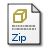# Approximating the set of pareto-optimal solutions in both the decision and objective spaces by an estimation of distribution algorithm

Zhou, A and Zhang, Q and Jin, Y (2009) 'Approximating the set of pareto-optimal solutions in both the decision and objective spaces by an estimation of distribution algorithm.' IEEE Transactions on Evolutionary Computation, 13 (5). 1167 - 1189. ISSN 1089-778XPDF (Paper) ces485.pdf Restricted to Repository staff only Download (14MB)Archive (ZIP) (C++ code) mmea(20090630).rar Restricted to Repository staff only Download (512kB)

## Abstract

Most existing multiobjective evolutionary algorithms aim at approximating the Pareto front (PF), which is the distribution of the Pareto-optimal solutions in the objective space. In many real-life applications, however, a good approximation to the Pareto set (PS), which is the distribution of the Pareto-optimal solutions in the decision space, is also required by a decision maker. This paper considers a class of multiobjective optimization problems (MOPs), in which the dimensionalities of the PS and the PF manifolds are different so that a good approximation to the PF might not approximate the PS very well. It proposes a probabilistic model-based multiobjective evolutionary algorithm, called MMEA, for approximating the PS and the PF simultaneously for an MOP in this class. In the modeling phase of MMEA, the population is clustered into a number of subpopulations based on their distribution in the objective space, the principal component analysis technique is used to estimate the dimensionality of the PS manifold in each subpopulation, and then a probabilistic model is built for modeling the distribution of the Pareto-optimal solutions in the decision space. Such a modeling procedure could promote the population diversity in both the decision and objective spaces. MMEA is compared with three other methods, KP1, Omni-Optimizer and RM-MEDA, on a set of test instances, five of which are proposed in this paper. The experimental results clearly suggest that, overall, MMEA performs significantly better than the three compared algorithms in approximating both the PS and the PF. © 2009 IEEE.

Item Type: Article Q Science > QA MathematicsQ Science > QA Mathematics > QA75 Electronic computers. Computer science Faculty of Science and Health > Computer Science and Electronic Engineering, School of Jim Jamieson 13 Jan 2012 11:21 17 Oct 2019 14:17 http://repository.essex.ac.uk/id/eprint/1972View Item